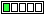All about flooble | fun stuff | Get a free chatterbox | Free JavaScript | Avatarsperplexus dot info00 Separate (Posted on 2003-10-10)Prove that the numbers that begin with 1 and end with 1, with any number of 2s in the middle, and all 1s and 2s separated by 00 are composite.

For example:
1002001
1002002001,
1002002002001,
1002002002002001

 See The Solution Submitted by Gamer Rating: 3.0000 (7 votes)Comments: ( Back to comment list | You must be logged in to post comments.)Puzzle Solution Comment 13 of 13 |We know that,
a^x + 2*a^(x-1) + 2*a^(x-2)+ ......+ 2a + 1
= (a+1)(a^(x-1) + a^(x-2)+ ......+ a + 1)

Substituting a = 1000, we have:

1000^x + 2*1000^(x-1) + 2*1000^(x-2)+ ......+ 2*1000 + 1
= 1001*(1000^(x-1) + 1000^(x-2)+ ......+ 1000 + 1)

Consequently, all the numbers having the given form is always divisible by 1001, and therefore composite.

 Posted by K Sengupta on 2007-11-29 04:47:54Please log in:

 Search: Search body:
Forums (0)